Thank you for visiting nature.com. You are using a browser version with limited support for CSS. To obtain the best experience, we recommend you use a more up to date browser (or turn off compatibility mode in Internet Explorer). In the meantime, to ensure continued support, we are displaying the site without styles and JavaScript.

# Efficient embedding of complex networks to hyperbolic space via their Laplacian

## Abstract

The different factors involved in the growth process of complex networks imprint valuable information in their observable topologies. How to exploit this information to accurately predict structural network changes is the subject of active research. A recent model of network growth sustains that the emergence of properties common to most complex systems is the result of certain trade-offs between node birth-time and similarity. This model has a geometric interpretation in hyperbolic space, where distances between nodes abstract this optimisation process. Current methods for network hyperbolic embedding search for node coordinates that maximise the likelihood that the network was produced by the afore-mentioned model. Here, a different strategy is followed in the form of the Laplacian-based Network Embedding, a simple yet accurate, efficient and data driven manifold learning approach, which allows for the quick geometric analysis of big networks. Comparisons against existing embedding and prediction techniques highlight its applicability to network evolution and link prediction.

## Introduction

The gradual addition of nodes and edges to a network, a common representation of the relationships between complex system components, imprints valuable information in its topology. Consequently, development of techniques to mine the structure of networks is crucial to understand the factors that play a role in the formation of their observable architecture.

Strong clustering and scale-free node degree distribution, properties common to most complex networks, have served as the basis for the establishment of tools for link prediction1, community detection2, identification of salient nodes3 and so on. In addition, several models that aim to mimic the evolution and formation of networks with the above-mentioned characteristics have been introduced4. Of special interest are a series of models that assume the existence of a hidden geometry underlying the structure of a network, shaping its topology5,6,7,8,9,10,11,12 (we refer the reader to13 for an extensive review on the subject). This is justified by the fact that complex networks possess characteristics commonly present in geometric objects, like scale invariance and self-similarity7,14,15,16.

One of such models is the so-called Popularity-Similarity (PS) model, which sustains that clustering and hierarchy are the result of an optimisation process involving two measures of attractiveness: node popularity and similarity between nodes12. Popularity reflects the property of a node to attract connections from other nodes over time and it is thus associated with a node’s seniority status in the system. On the other hand, nodes that are similar have a high likelihood of getting connected, regardless of their rank.

The PS model has a geometric interpretation in hyperbolic space, where the trade-off between popularity and similarity is abstracted by the hyperbolic distance between nodes9,12. Short hyperbolic distances between them correlate strikingly well with high probabilities of link formation12. This means that mapping a network to hyperbolic space unveils the value of the variables in charge of shaping its topology (popularity and similarity in this case), allowing for a better understanding of the dynamics accountable for the system’s growth process.

Current efforts to infer the hyperbolic geometry of complex networks bet for a Maximum Likelihood Estimation (MLE) approach, in which the space of PS models with the same structural properties as the network of interest is explored, in search for the one that better fits the network topology12,17,18. This search is computationally demanding, which means that these methods require of correction steps or heuristics in order to make them suitable for big networks18.

In this paper, a different strategy is followed. Inspired by the well-established field of non-linear dimensionality reduction in Machine Learning19, an adaptation of the Laplacian Eigenmaps algorithm, introduced by Belkin and Niyogi for the low-dimensional representation of complex data20, is put forward for the embedding of complex networks into the two-dimensional hyperbolic plane. The proposed approach is based on the approximate eigen-decomposition of the network’s Laplacian matrix, which makes it quite simple yet accurate and efficient, allowing for the analysis of big networks in a matter of seconds. Furthermore, it is data driven, which means that no assumptions are made about the model that constructed the network of interest. Finally, benchmarking of the method against existing embedding and prediction techniques highlights the advantages of using it for the prediction of new links between nodes and for the study of network evolution.

## Results

### Preliminaries and the proposed method

In this paper, only undirected, unweighted, single-component networks are considered, as the proposed embedding approach is only applicable to networks with these properties20. Moreover, these networks are assumed to be scale-free (with scaling exponent γ  [2, 3]) and with clustering coefficientsignificantly larger than expected by chance. These networks are graphs G = (V, E) with N = |V| nodes and L = |E| edges connecting them. An undirected, unweighted graph can be represented by an N × N adjacency matrix Ai,j = Aj,i i, j, whose entries are 1 if there is an edge between nodes i and j and 0 otherwise. The graph Laplacian is a transformation of A given by L = D − A, where D is a matrix with the node degrees on its diagonal and 0 elsewhere.

Let us now consider a real-valued function on the set of network nodes,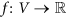, which assigns a real number f (i) to each graph node. If the Laplacian acts as an operator over this function, Lf (i) = ∑jAi,j(f (i) − f (j)), one can see that it is giving information about how the value of f for each node i compares to that of its neighbours j21. This is the discrete analogue of the Laplace operator in vector calculus and its generalisation in differential geometry, the Laplace-Beltrami operator20, which measure how much the curvature of a surface is changing at a given point. This is more evident if one thinks of a function as being approximated by a graph, such that nodes have more edges where the value of the Laplacian is greater (see Fig. 1).

In particular, embedding of the network to the two-dimensional hyperbolic plane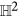, represented by the interior of a Euclidean circle9, is given by the N × 2 matrix Y = [y1, y2] where the ith row, Yi, provides the embedding coordinates of node i. Using the Laplacian operator (see above), this corresponds to minimising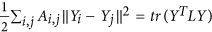, which reduces to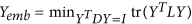with D as defined above, I the identity matrix, MT the transpose of M and tr(M) the trace of M. Finally, Yemb, the matrix that minimises this objective function, is formed by the two eigenvectors with smallest non-zero eigenvalues that solve the generalised eigenvalue problem LY = λDY20 (see Section 1 in the Supplementary Information for a detailed justification of this embedding approach).

In the context of manifold learning, most algorithms rely on the construction of a mesh or network over the high-dimensional manifold containing the samples of interest19,22. When pairwise distances between samples are computed, they correspond to shortest-paths over the constructed network, allowing for a better preservation of the sample relationships when the data is embedded to low dimensions19,20,22,23. If there is really a hyperbolic geometry underlying a complex network, it should lie on a hyperbolic plane, with nodes drifting away from the space origin. If the network itself is seen as the mesh that connects samples (nodes in this case) that are close to each other12, it can be used as in manifold learning to recover the hyperbolic coordinates of its nodes. Connected pairs of nodes in the network should be very close to each other in the target, low-dimensional space (hence the minimisation problem presented above) and, consequently, their angular separation (governed by their similarity dimension according to the PS model) should also be small. Figure 2a shows that, if the described embedding approach is employed, this is indeed the case for an artificial network generated with the PS model (see the Methods for more details).

As a result, to complete the mapping to, angular node coordinates are obtained via θ = arctan(y2/y1) and radial coordinates (abstracted by the popularity or seniority dimension in the PS model) are chosen so as to resemble the rank of each node according to its degree. This is achieved via ri = 2β ln(i) + 2(1 − β) ln(N), where nodes i = {1, 2, …, N} are the network nodes sorted decreasingly by degree and β = 1/(γ − 1)9,12 (see Fig. 2b and the Methods for further details).

This strategy is valid, because the native representation of, in which the hyperbolic space is contained in a Euclidean disc and Euclidean and hyperbolic distances from the origin are equivalent, is a conformal model. This means that Euclidean angular separations between nodes are also equivalent to hyperbolic ones9. On the other hand, the radial arrangement of nodes corresponds to a quasi-uniform distribution of radial coordinates in the disc9. It is also important to mention that, due to rotational invariance of distances, the set of hyperbolic coordinates responsible for the edges observed in a network is not unique (see Fig. 2b). Therefore, the goal of the proposed technique is not to find a specific set of coordinates, but the one that corresponds better with the hyperbolic, distance-dependent connection probabilities that produce the network of interest.

The network embedding approach described in this section and in Table 1 is hereafter referred to as Laplacian-based Network Embedding (LaBNE).

### Benchmarking LaBNE

In order to test the ability of LaBNE to infer the hyperbolic geometry of complex networks, artificial networks were generated using the PS model. This model allows for the construction of networks with known hyperbolic coordinates for each of its N nodes and target average node degree 2m, scaling exponent γ and clustering coefficient. The latter is controlled by the network temperature T, is reduced almost linearly with T and is the strongest possible at T = 09 (see Methods for more details). One hundred synthetic networks were grown for each combination of parameters T = {0, 0.3, 0.6, 0.9}, 2m = {4, 6, 8, 10} and γ = {2.25, 2.50, 2.75}; the number of nodes N was fixed to 500. These networks were then mapped towith LaBNE and Pearson correlations between the inferred hyperbolic distances and the ones measured with the real node coordinates were computed and averaged across the one hundred networks for each parameter configuration. This same procedure was followed for the most recent and fastest version of HyperMap, a MLE method for network embedding to hyperbolic space that finds node coordinates by maximising the likelihood that the network is produced by the PS model17,18 (see Methods for details).

Figure 3a shows how the average correlation for each parameter combination, obtained by LaBNE, is as high or higher than the one obtained by HyperMap. This is especially evident in dense, strongly clustered networks (i.e. networks with large 2m and T → 0, which implies high). These results are supported by the fact that angular coordinates inferred by LaBNE are closer to the angles from the generated PS networks, compared to the ones inferred by HyperMap (see Figure S1).

To provide an in-depth analysis of the differences in time performance of the two algorithms, an experiment similar to the one presented in Fig. 3a was carried out, but this time parameter T was fixed to 0 and the network size changed as N = {250, 500, 750, 1000}. Figure 3b depicts that the fold-change from the average time needed by LaBNE to embed each of the one hundred networks per parameter configuration to the average time needed by HyperMap to perform the same task is very big, the latter being 500 times slower than LaBNE for the smallest networks. These results highlight one of the great benefits of LaBNE, its time performance, which stems from its computational complexity of O(kN2) with k = 3. In a network with a single connected component, the smallest eigenvalue of L is always 021 and its corresponding eigenvector is discarded. The next two, which correspond to the smallest non-zero eigenvalues, form the resulting node coordinates. Since k is always constant, LaBNE can be considered a O(N2) algorithm.

In spite of the version of HyperMap considered here being a O(N2) method as well18 (see Methods for details), Fig. 3b shows that it is slower than LaBNE. This is because the heuristic used to speed up this algorithm is not actually applied to all N nodes in the network of interest, but only to those with degrees smaller than a parameter kspeedup18 (see Methods for details). The larger the value of this parameter, the faster the algorithm, but also the higher the impact on its accuracy. It was set to 10 throughout this paper, which produced good results in a reasonable amount of time. On the other hand, LaBNE takes advantage of the efficiency and portability of the R package igraph24 and its interface with high-performance subroutines designed to solve large scale eigenvalue problems25.

### Hyperbolic embedding for link prediction in real networks

Given the accuracy and time performance achieved by LaBNE and considering that short hyperbolic distances correlate well with high probabilities of connection between nodes12, LaBNE was used to infer the hyperbolic coordinates for the nodes of three real networks (see Table 2 and Methods) to then carry out link prediction. As discussed in the previous section, Figure 3 shows that the accuracy of LaBNE is higher for networks with more topological information, i.e. with more edges between nodes, which occurs at high average node degrees (2m) and low temperatures (which imply high clustering). Consequently, the three real networks analysed here were chosen with the aim to investigate the performance of LaBNE in the low, medium and high clustering coefficient scenarios (see Table 2). Furthermore, these network datasets represent complex systems from different domains: the high quality human protein interaction network (PIN) models the relationships between proteins within the human cell (low), in the Pretty-Good-Privacy network (PGP) users share encryption keys with people they trust (medium) and the autonomous systems Internet (ASI) corresponds to the communication network between groups of routers (high, see the Methods for more details).

The above-described evaluation framework comes, however, with a critical caveat. Pruning edges at random can remove important information from the observable network topology in unpredictable ways and this most certainly affects link predictors differently26. To avoid this problem, historical data of the evolution of the network must be used to test the ability of a link predictor to assign high likelihood scores to edges in a network Gt + 1 that are not yet present in a snapshot Gt, to which the predictor is applied. For the ASI27 and the PGP, temporal snapshots of their topology were available and this evaluation method was used (see networks with subscript t + 1 in Table 2). For the PIN28, it was necessary to resort to the so-called Guilt-by-association Principle29, which states that two proteins are highly likely to interact if they are involved in the same biological process. In this scenario, link predictors are applied to the observable protein network and discrimination between good and bad candidate interactions is based on a stringent cut-off on a measure of the similarity between the biological processes of the non-adjacent proteins (see the Methods for more details).

Figure 4a–c shows the performance in link prediction of LaBNE, HyperMap and a set of neighbourhood-based link predictors that are commonly considered for benchmarking in this context (see Methods). As expected from the results on artificial networks (Fig. 3a), the performance of both LaBNE and HyperMap improves as clustering increases. The worst results are thus obtained in the PIN (Fig. 4a). However, LaBNE is the only prediction technique that allows for more Recall without sacrificing as much Precision as the others. The performance of HyperMap in this network is bad because, as it can be seen in Fig. 3a and S1b, its application should be restricted to highly clustered networks. In the other two cases (Fig. 4b,c), LaBNE is the second best performing method in terms of area under the Precision-Recall curve, only slightly behind HyperMap. These are very good results considering that LaBNE can obtain reliable link predictions in these big networks in a matter of seconds, while the current implementation of the most recent and fastest version of HyperMap requires days to produce the results (see Table 2).

Regarding the performance of the neighbourhood-based link predictors, it is important to highlight their good Precision at low Recall levels. This is mainly due to the fact that they are only able to assign meaningful scores to node pairs separated by at most two hops, which is a very small fraction of all possible candidate edges. The rest obtain the exact same score, which prevents from differentiating between good and bad candidate interactions and results in the rapid drop of Precision observed in Fig. 4a–c.

### Hyperbolic embedding for real network evolution analysis

In the PS model, radial coordinates are directly proportional to node birth-times, i.e. if a node i is close to the origin of the hyperbolic circle containing the network (ri → 0), it means it was born early in the evolution of the complex system12. To test whether this is the case in the most recent temporal snapshot of the three real networks considered (PIN, PGP and ASI), node radial coordinates inferred by LaBNE were compared to actual node birth-times (see the Methods for details on how birth-times are defined for each real network; HyperMap was not used here as it practically produces the same radial coordinates as LaBNE).

Figure 5a–c shows that in the three cases, nodes that are close to the centre of the hyperbolic space are older than those located in its periphery. Even all nodes from the first network snapshot in the PGP and ASI, which represent a mix of nodes that appeared at that time and nodes from older, not available time-points, possess small radial coordinates (Fig. 5b,c).

These are very important results, because they exhibit the close relationship between node popularity and seniority in networks of very different objects and time scales. The results obtained with LaBNE suggest that, even when the identity of the network nodes is unknown, one can have an idea of their history in the system under study, based merely on their degree and, consequently, their inferred radial positions.

## Conclusions

Scale-invariance, self-similarity and strong clustering, properties present in complex systems and geometric objects alike, have led to the proposal that the network representations of the former lie on a geometric space where distance constraints play important roles in the formation of links between system components8,9,12,30. One of such proposals advocates for the hyperbolic space as a good candidate to host complex networks, given that their skeletons (trees abstracting their underlying hierarchical structure) require an exponential space to branch and only hyperbolic spaces expand exponentially9.

In consequence, efficient and accurate methods to embed networks to hyperbolic space are needed. In this article, a novel approach to perform this task is proposed: the Laplacian-based Network Embedding or LaBNE. Since it is based on a transformation of the adjacency matrix representation of a network, namely the graph Laplacian, it highly depends on topological information to carry out good embeddings. This was confirmed when applied to artificial and real networks with differing structural characteristics. The higher the average node degree (2m) and clustering coefficient () of a network, the better the results achieved by LaBNE. Nevertheless, its low computational complexity allows for the study of the hyperbolic geometry of big networks in a matter of seconds. This means that LaBNE is suited to draft a geometric configuration of a network, which can then be used by more involved and time consuming techniques, thus reducing the space of possible node coordinates they have to explore.

Notwithstanding the fact that techniques for embedding networks to generic low-dimensional spaces have been proposed to facilitate their visualisation and analysis19,20,22,23,30,31,32,33, it is important to stress that LaBNE deals specifically with the embedding to the two-dimensional hyperbolic plane. This space has been shown to provide an accurate reflection of the geometry of real networks9,12 (see Figs 4 and 5) and allows for their visual inspection in two or three dimensions (see Figure S2). However, LaBNE does not make any a priori assumption about the model or mechanism that led to the formation of the network of interest. Thus, the distance-dependent connection probabilities resulting from the mapping toserve as the basis to determine if such a space is suitable for the network or not. For example, networks grown with the Barabasi-Albert model14 are infinite-dimensional hyperbolic networks34, but short distances between their nodes, measured with the coordinates inferred by LaBNE or HyperMap in, are not indicative of link formation in this space (see Figure S3). The in-depth study of networks with high-dimensional latent spaces or their embedding to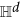(with d > 2) are of great interest, but beyond the scope of this article.

Finally, although this work did not intend to provide an extensive comparison between link predictors or a thorough analysis of the evolution of real networks, it is important to note that LaBNE performed very well in these two type of studies when applied to a biological, a social and a technological network. These represent a few example scenarios in which the inference of the hyperbolic geometry underlying a network could be useful.

## Methods

### The PS model

The PS model12 on the hyperbolic plane of curvature K = −1 is formulated as follows: (1) initially the network is empty; (2) at time t ≥ 1, a new node t appears at coordinates (rt, θt) with rt = 2 lnt and θt uniformly distributed on [0, 2π] and every existing node s < t increases its radial coordinate according to rs(t) = β rs + (1 − β) rt with β = 1/(γ − 1)  [0, 1]; (3) new node t picks a randomly chosen node s < t that is not already connected to it and links with it with probability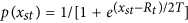, where parameter T, the network temperature, controls the network’s clustering coefficient,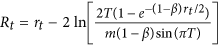is the current radius of the hyperbolic circle containing the network, xst = rs + rt + 2ln(θst/2) is the hyperbolic distance between nodes s and t and θst is the angle between the nodes; (4) repeat step 3 until node t gets connected to m different nodes; (5) repeat steps 1–4 until the network is comprised of N nodes. Note that if T → 0,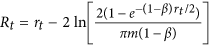. In addition, if β = 1/(γ − 1) = 1, existing nodes do not change their radial coordinates and Rt = 2lnt.

### Radial arrangement of nodes in LaBNE

As described above, new nodes in the PS model acquire radial coordinates rt = 2lnt that depend on their birth-time t. This means that the probability of finding a node that is close to the centre of the hyperbolic circle containing the growing network, is exponentially lower than the probability to find a node on the periphery. When a new node is added to the system and the existing ones change their radial position according to rs(t) = β rs + (1 − β) rt, where β = 1/(γ − 1), their seniority is attenuated by increasing their distances to every newly added node12. Consequently, the N angular coordinates found by LaBNE are complemented with the nodes’ radial coordinates obtained via ri = 2β ln(i) + 2(1 − β) ln(N), where nodes i = {1, 2, …, N} are the network nodes sorted decreasingly by degree.

### HyperMap

HyperMap17 is a Maximum Likelihood Estimation method to embed a network to hyperbolic space. It finds node coordinates by replaying the network’s hyperbolic growth and, at each step, maximising the likelihood that it was produced by the PS model17. For embedding to the hyperbolic plane of curvature K = −1 it works as follows: (1) nodes are sorted decreasingly by degree and labelled i = {1, 2, …, N} from the top of the sorted list; (2) node i = 1 is born and assigned radial coordinate r1 = 0 and a random angular coordinate θ1 [0, 2π]; (3) for each node i = {2, 3, …, N}: (3.1) node i is born and assigned radial coordinate ri = 2lni; (3.2) the radial coordinate of every existing node j < i is increased according to rj(i) = β rj + (1 − β) ri; (3.3) node i is assigned the angular coordinate θi maximising the likelihood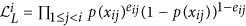. β and p(xij) are defined as in the PS model and eij is 1 if nodes i and j are connected and 0 otherwise. The maximisation of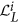is performed numerically by trying different values of θ in [0, 2π], separated by intervals Δθ = 1/i and then choosing the one that produces the greatest.

Since the angular coordinates yielded by this link-based likelihood are not very accurate for small i (i.e. for high degree nodes)17, the fast version of HyperMap used in this paper uses information on the final number of common neighbours between these old nodes via the maximisation of the log-likelihood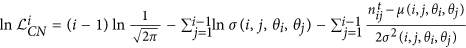, where μ is the mean number of common neighbours nij between i and j and σ2 is the associated variance18. This hybrid version of HyperMap is O(N3) and to speed it up, Papadopoulos and colleagues resort to the following heuristic: for nodes i with degree ki < kspeedup, an initial estimate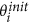of their angular coordinate is computed by considering only the previous nodes j < i that are their neighbours; these estimates are then refined, searching for the final θi within a small region around. The fast hybrid version of HyperMap with kspeedup = 10 is the one used throughout this work. We refer the reader to18 for more details on the speed-up heuristic and the derivation of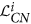. Finally, even when correction steps can be used together with the fast hybrid HyperMap, their effect on this method has been reported not to be significant18 and they are not considered here.

### Network datasets

For the three network datasets used in this paper, self-loops and multiple edges were discarded and only the largest connected component was considered.

The high-quality protein interaction network (PIN) is a stringent subset of the Human Integrated Protein-Protein Interaction rEference (HIPPIE)28. HIPPIE retrieves interactions between human proteins from major expert-curated databases and calculates a score for each one, reflecting its combined experimental evidence. This score is a function of the number of studies supporting the interaction, the quality of the experimental techniques used to measure it and the number of organisms in which the orthologs of the interacting human proteins interact as well. In this paper, only interactions with confidence scores ≥0.73 (the upper quartile of all scores) in release 1.7 were considered. The raw version of this network is available at http://cbdm-01.zdv.uni-mainz.de/mschaefer/hippie/download.php. To determine the birth-time of the PIN nodes, proteins from the manually curated database SwissProt were clustered based on near full-length similarity and/or high threshold of sequence identity using FastaHerder235. If proteins from two evolutionarily distant organisms are present in one cluster, this suggests that the protein family is ancient. The minimum common taxonomy from all proteins that are part of a cluster was taken as an indication of the cluster’s age. Each node of the PIN was assigned to one of the following age clusters: Tree of Life Root, Metazoa, Chordata, Mammalia, Euarchontoglires or Primates/Human.

Pretty-Good-Privacy (PGP) is a data encryption and decryption program for secure data communication. In a PGP web of trust, each user (node) knows the public key of a group of people he trusts. When user A wants so send information to user B, this information is encrypted with B’s public key and signed with A’s private key. When B receives the information, he verifies that the message is coming from one of the users he trusts and decrypts it with his private key36. This encryption and decryption event, forms a directed link between users A and B. In this article, however, the edge directionality of this network is not considered. This is not a problem for the interpretation of the network if we assume that by sharing a key, two users reciprocally endorse their trust in each other12. The four temporal snapshots of the undirected PGP network used here, which were collected by Jörgen Cederlöf 37, were used to assign a birth-time for each user based on the snapshot in which he first appeared. The snapshots correspond to April and October 2003, December 2005 and December 2006. The raw PGP data is available at http://www.lysator.liu.se/jc/wotsap/wots2/.

The autonomous systems Internet (ASI) corresponds to the communication network between IPv4 Internet sub-graphs comprised of routers, as collected by the Center for Applied Internet Data Analysis27. The six available network snapshots, spanning the period from September 2009 to December 2010 in 3-month intervals, were used to determine the birth-time of each autonomous system based on the snapshot in which it first appeared. These Internet topologies are available for download at https://bitbucket.org/dk-lab/2015_code_hypermap.

The performance of LaBNE and HyperMap in link prediction was compared to that of reference neighbourhood-based link predictors. They receive this name because the scores they produce are usually based on how much overlap there is between the neighbourhoods of non-adjacent pairs of nodes in a network. These unlinked nodes are often called seed nodes.

The simplest predictor considered was the Common Neighbours (CN) index, which just counts the number of common neighbours between seed nodes38. The other indices examined were the Dice Similarity (DS), which is one of the possible normalisations of CN39; the Adamic and Adar (AA) index, which assigns higher likelihood scores to seed nodes whose CNs do not interact with other components40; and the Preferential Attachment index (PA), which is simply the degree product of the seed nodes38. The formulae for these indices are, respectively: CN(x, y) = |Γ(x) ∩ Γ(y)|, DS(x, y) = 2CN(x, y)/(|Γ(x)| + |Γ(y)|),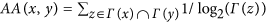and PA(x, y) = |Γ(x)||Γ(y)|, where Γ(x) is the set of neighbours of x and |Γ(x)| is the set cardinality.

The embedding- and neighbourhood-based predictors were applied to all the seed nodes of a network snapshot Gt, these node pairs were later sorted from best to worst score. This sorted list was scanned with a moving score threshold from top to bottom to compute the proportion of candidate interactions taken that coincide with the set of new edges in Gt+1 (Precision) and the proportion of candidate interactions not taken at each threshold but that belong to the set of new edges present in Gt+1 (Recall). This allowed for the construction of a Precison-Recall curve for each of the predictors considered.

For the PGP, Gt has 14367 nodes and 37900 edges (snapshot from April 2003), while Gt+1 has 31524 nodes and 168559 edges (all the nodes and edges from April 2003 to December 2006). Note, however that only the 62547 new links between the same set of 14367 nodes present in Gt are considered.

For the ASI, Gt has 24091 nodes and 59531 edges (snapshot from September 2009), while Gt+1 has 34320 nodes and 128839 edges (all the nodes and edges from September 2009 to December 2010). Note, however that only the 48119 new links between the same set of 24091 nodes present in Gt are considered.

For the PIN, it was necessary to follow a different procedure, as edge timestamps are not available for this network. When the performance of a link predictor is assessed in protein networks, researchers have opted for using Gene Ontology (GO) similarities to discriminate between good and bad candidate interactions30,32,41,42. This is based on the Guilt-by-association Principle, which states that if two proteins are involved in similar biological processes, they are more likely to interact29. So, the link predictors were applied to the non-adjacent protein pairs in the observable network topology of the PIN and then sorted from best to worst score. The GO similarity of the top 10% candidate links was then computed, together with the proportion of protein pairs with similarities ≥0.75. This percentage corresponds to the precision of the link predictors reported for the PIN.

To compute GO similarities the R package GOSemSim was utilised43. Although this package provides different indices to measure similarities between proteins, Wang’s index was used because it was formulated specifically for the GO44. GO similarities on the high end of the range [0, 1] are normally good indicators of a potential protein interaction44. However, a threshold of 0.544 was preferred in this study, as it corresponds to the upper quartile of all the GO similarities of connected protein pairs in the PIN.

### Hardware used for experiments

All the experiments presented in this paper were executed on a Lenovo ThinkPad 64-bit with 7.7 GB of RAM and an Intel Core i7-4600U CPU @ 2.10 GHz × 4, running Ubuntu 14.04 LTS. The only exception were the link prediction experiments, which were executed on nodes with 100 GB of RAM, within the Mogon computer cluster at Johannes Gutenberg Universität in Mainz.

### Availability

R implementations of the PS model and LaBNE are available at http://www.greg-al.info/code. The network data used in this paper are also available at the same website. The C++ implementation of the fast version of HyperMap used in this paper is available at https://bitbucket.org/dk-lab/2015_code_hypermap.

How to cite this article: Alanis-Lobato, G. et al. Efficient embedding of complex networks to hyperbolic space via their Laplacian. Sci. Rep. 6, 30108; doi: 10.1038/srep30108 (2016).

## References

1. Lü, L. & Zhou, T. Link prediction in complex networks: a survey. Physica A 390, 1150–1170 (2011).

2. Harenberg, S. et al. Community detection in large-scale networks: a survey and empirical evaluation. Wiley Interdiscip. Rev. Comput. Stat. 6, 426–439 (2014).

3. Opsahl, T., Agneessens, F. & Skvoretz, J. Node centrality in weighted networks: generalizing degree and shortest paths. Soc. Networks 32, 245–251 (2010).

4. Shore, J. & Lubin, B. Spectral goodness of fit for network models. Soc. Networks 43, 16–27 (2015).

5. Dall, J. & Christensen, M. Random geometric graphs. Phys. Rev. E 66, 016121 (2002).

6. Aste, T., Di Matteo, T. & Hyde, S. Complex networks on hyperbolic surfaces. Physica A 346, 20–26 (2005).

7. Serrano, M. A., Krioukov, D. & Boguñá, M. Self-similarity of complex networks and hidden metric spaces. Phys. Rev. Lett. 100, 078701 (2008).

8. Boguñá, M., Krioukov, D. & Claffy, K. C. Navigability of complex networks. Nat. Phys. 5, 74–80 (2009).

9. Krioukov, D., Papadopoulos, F., Kitsak, M., Vahdat, A. & Boguñá, M. Hyperbolic geometry of complex networks. Phys. Rev. E 82, 036106 (2010).

10. Ferretti, L. & Cortelezzi, M. Preferential attachment in growing spatial networks. Phys. Rev. E 84, 016103 (2011).

11. Aste, T., Gramatica, R. & Di Matteo, T. Exploring complex networks via topological embedding on surfaces. Phys. Rev. E 86, 036109 (2012).

12. Papadopoulos, F., Kitsak, M., Serrano, M. A., Boguñá, M. & Krioukov, D. Popularity versus similarity in growing networks. Nature 489, 537–540 (2012).

13. Barthélemy, M. Spatial networks. Phys. Rep. 499, 1–101 (2011).

14. Barabãsi, A.-L. & Albert, R. Emergence of scaling in random networks. Science 286, 509–512 (1999).

15. Song, C., Havlin, S. & Makse, H. A. Origins of fractality in the growth of complex networks. Nat. Phys. 2, 275–281 (2006).

16. Goh, K.-I., Salvi, G., Kahng, B. & Kim, D. Skeleton and fractal scaling in complex networks. Phys. Rev. Lett. 96, 018701 (2006).

17. Papadopoulos, F., Psomas, C. & Krioukov, D. Network mapping by replaying hyperbolic growth. IEEE ACM T. Network. 23, 198–211 (2015).

18. Papadopoulos, F., Aldecoa, R. & Krioukov, D. Network geometry inference using common neighbors. Phys. Rev. E 92, 022807 (2015).

19. Cayton, L. Algorithms for manifold learning. UCSD tech report CS2008–0923, 1–17 (2005). URL http://www.lcayton.com/resexam.pdf. Last visited: 2016-03-30.

20. Belkin, M. & Niyogi, P. Laplacian eigenmaps and spectral techniques for embedding and clustering. Adv. Neur. In. 14, 585–591 (2001).

21. Brouwer, A. E. & Haemers, W. H. Spectra of graphs (Springer-Verlag: New York, 2012).

22. Zemel, R. S. & Carreira-Perpiñán, M. A. Proximity graphs for clustering and manifold learning. Adv. Neur. In. 17, 225–232 (2004).

23. Tenenbaum, J. B. A Global Geometric Framework for Nonlinear Dimensionality Reduction. Science 290, 2319–2323 (2000).

24. Csardi, G. & Nepusz, T. The igraph software package for complex network research. InterJournal Compl. Syst. 1695, 1695 (2006).

25. Lehoucq, R., Sorensen, D. & Yang, C. ARPACK Users’ Guide (Society for Industrial and Applied Mathematics, 1998).

26. Yang, Y., Lichtenwalter, R. N. & Chawla, N. V. Evaluating link prediction methods. Knowl. Inf. Syst. 45, 751–782 (2014).

27. Claffy, K., Hyun, Y., Keys, K., Fomenkov, M. & Krioukov, D. Internet mapping: from art to science. 205–211 (IEEE, 2009).

28. Schaefer, M. H. et al. HIPPIE: integrating protein interaction networks with experiment based quality scores. PLoS ONE 7, e31826 (2012).

29. Oliver, S. Guilt-by-association goes global. Nature 403, 601–603 (2000).

30. Cannistraci, C. V., Alanis-Lobato, G. & Ravasi, T. Minimum curvilinearity to enhance topological prediction of protein interactions by network embedding. Bioinformatics 29, i199–i209 (2013).

31. Kuchaiev, O., Rašajski, M., Higham, D. J. & Pržulj, N. Geometric De-noising of Protein-Protein Interaction Networks. PLoS Comput. Biol. 5, e1000454 (2009).

32. You, Z.-H. H., Lei, Y.-K. K., Gui, J., Huang, D.-S. S. & Zhou, X. Using manifold embedding for assessing and predicting protein interactions from high-throughput experimental data. Bioinformatics 26, 2744–2751 (2010).

33. Newman, M. & Peixoto, T. P. Generalized communities in networks. Phys. Rev. Lett. 115, 088701 (2015).

34. Ferretti, L., Cortelezzi, M. & Mamino, M. Duality between preferential attachment and static networks on hyperbolic spaces. Europhys. Lett. 105, 38001 (2014).

35. Mier, P. & Andrade-Navarro, M. A. FastaHerder2: four ways to research protein function and evolution with clustering and clustered databases. J. Comput. Biol. 23, 270–278 (2016).

36. Schneier, B. Applied cryptography (John Wiley & Sons, 1996).

37. Cederlöf, J. The OpenPGP web of trust (2003). URL http://www.lysator.liu.se/jc/wotsap/wots2/. Last visited: 2015-09-08.

38. Newman, M. Clustering and preferential attachment in growing networks. Phys. Rev. E 64, 1–4 (2001).

39. Dice, L. R. Measures of the amount of ecologic association between species. Ecology 26, 297–302 (1945).

40. Adamic, L. & Adar, E. Friends and neighbors on the web. Soc. Networks 25, 211–230 (2003).

41. Chen, J., Hsu, W., Lee, M. L. & Ng, S.-K. Increasing confidence of protein interactomes using network topological metrics. Bioinformatics 22, 1998–2004 (2006).

42. Cannistraci, C. V., Alanis-Lobato, G. & Ravasi, T. From link-prediction in brain connectomes and protein interactomes to the local-community-paradigm in complex networks. Sci. Rep. 3, 1613 (2013).

43. Yu, G. et al. GOSemSim: an R package for measuring semantic similarity among go terms and gene products. Bioinformatics 26, 976–978 (2010).

44. Wang, J., Du, Z., Payattakool, R., Yu, P. & Chen, C.-F. A new method to measure the semantic similarity of GO terms. Bioinformatics 23, 1274–1281 (2007).

## Acknowledgements

The authors would like to thank Carlo V. Cannistraci and Josephine Thomas for useful comments and suggestions. In addition, the posts published by Alex Kritchevsky and Arpan Saha on http://www.quora.com/ were very important for a clear and intuitive presentation of the Laplacian as an operator. Finally, the authors also thank the Zentrum für Datenverarbeitung at the Johannes Gutenberg Universität in Mainz, which made the analysis of big networks possible in the Mogon computer cluster.

## Author information

Authors

### Contributions

G.A.-L. created LaBNE, designed, implemented and carried out the experiments. P.M. was in charge of the protein age assignment. M.A.A.-N. supervised the research. G.A.-L. wrote the manuscript, incorporating comments, contributions and corrections from P.M. and M.A.A.-N.

## Ethics declarations

### Competing interests

The authors declare no competing financial interests.

## Rights and permissions

Reprints and Permissions

• ### The inherent community structure of hyperbolic networks

• Bianka Kovács
•  & Gergely Palla

Scientific Reports (2021)

• ### Network geometry

• Marián Boguñá
• , Ivan Bonamassa
• , Manlio De Domenico
• , Shlomo Havlin
• , Dmitri Krioukov
•  & M. Ángeles Serrano

Nature Reviews Physics (2021)

• ### Optimisation of the coalescent hyperbolic embedding of complex networks

• Bianka Kovács
•  & Gergely Palla

Scientific Reports (2021)

• ### Hyperbolic node embedding for temporal networks

• Lili Wang
• , Chenghan Huang
• , Weicheng Ma
• , Ruibo Liu
•  & Soroush Vosoughi

Data Mining and Knowledge Discovery (2021)

• ### Reducing the complexity of financial networks using network embeddings

• M. Boersma
• , A. Maliutin
• , S. Sourabh
• , L. A. Hoogduin
•  & D. Kandhai

Scientific Reports (2020)